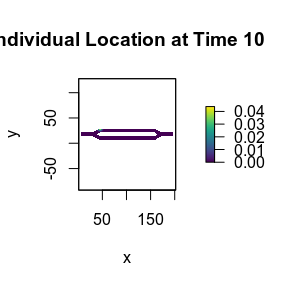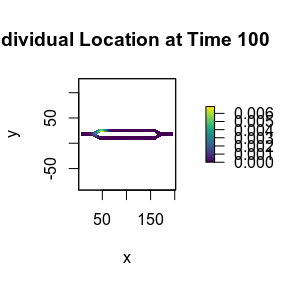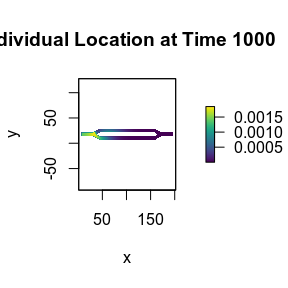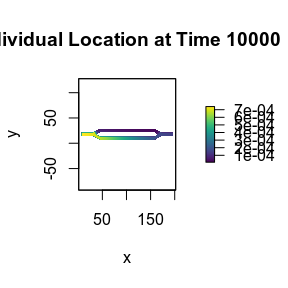# Temporal Analysis

## Introduction

This tutorial shows a simple example of visualizing one of the temporal metrics using the base plotting tools in R.

## Setup

# First step is to load the libraries. Not all of these libraries are stricly
# needed; some are used for convenience and visualization for this tutorial.
library("samc")
library("raster")
library("ggplot2")
library("viridis")

# "Load" the data. In this case we are using data built into the package.
# In practice, users will likely load raster data using the raster() function
# from the raster package.
res_data <- samc::ex_res_data
abs_data <- samc::ex_abs_data
occ_data <- samc::ex_occ_data

# Create a samc object using the resistance and absorption data. We use the
# recipricol of the arithmetic mean for calculating the transition matrix. Note,
# the input data here are matrices, not RasterLayers. If using RasterLayers, the
# latlon parameter must be set.
samc_obj <- samc(res_data, abs_data, tr_fun = function(x) 1/mean(x))

## Temporal Analysis

# Calculate the probabilities of where an individual starting at specific
# location will be for varying time steps. The starting location is going to
# be cell 1 in the landscape, which is the first non-NA cell going in a
# left-to-right then top-to-bottom order
time_steps <- c(10, 100, 1000, 10000)

for (ts in time_steps) {
dist <- distribution(samc_obj, origin = 1, time = ts)
dist_map <- map(samc_obj, dist)
plot(dist_map, main = paste("Individual Location at Time", ts), xlab = "x", ylab = "y", col = viridis(256))
}